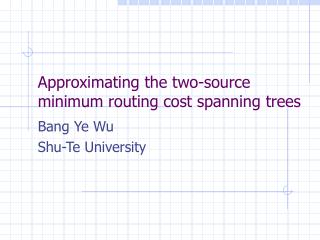DownloadDownload PresentationApproximating the two-source minimum routing cost spanning trees

# Approximating the two-source minimum routing cost spanning trees

Download Presentation## Approximating the two-source minimum routing cost spanning trees

- - - - - - - - - - - - - - - - - - - - - - - - - - - E N D - - - - - - - - - - - - - - - - - - - - - - - - - - -
##### Presentation Transcript

1. Approximating the two-source minimum routing cost spanning trees Bang Ye Wu Shu-Te University

2. Problem Definition • Input: an undirected graph with nonnegative edge lengths, two vertices as sources and all vertices as destinations • Output: a spanning tree such that the total distance from sources to destinations (including the sources) is minimum, that is, we want to minimize

3. Result • The NP-hardness of the problem. • A PTAS: for any e>0, the scheme finds a (1+ e)-approximation solution with time complexity

4. Related problems • OCT: SlijdT(i,j), arbitrary nonnegative requirements. • MRCT: requirement=1 • PROCT: lij=ri*rj, ri is nonnegative vertex weight • SROCT: lij=ri+rj • k-MRCT is a special case of SROCT. The sources have weight 1 and others have weight 0

5. OCT problems

6. Previous results

7. Future research • k-MRCT, fixed k and arbitrary k • K-OCT, fixed k and arbitrary k • On the plane